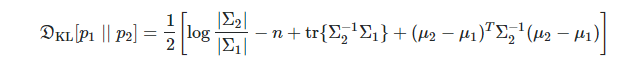# KL-divergence between two multivariate gaussian

I have two multivariate Gaussian distributions that I would like to calculate the kl divergence between them. each is defined with a vector of mu and a vector of variance (similar to VAE mu and sigma layer). What is the best way to calculate the KL between the two? Is this even doable? because I do not have the covariance matrix. If that is not doable, what if I take samples from both distributions and calculate the KL between the samplings? is that the right way of doing this? is there any build-in function for any of these calculations?

Hi,

Yes, this is the correct approach.
Just be aware that the input `a` must should contain `log-probabilities` and the target `b` should contain `probability`.

https://pytorch.org/docs/stable/nn.functional.html?highlight=kl_div#kl-div

By the way, PyTorch use this approach:

https://pytorch.org/docs/stable/distributions.html?highlight=kl_div#torch.distributions.kl.kl_divergence

Good luck
Nik

Thanks Nick for your input. I should restate my question. I have two multi-variate distributions each defined with “n” mu and sigma. Now I would like to do a KL between these two. Any idea how that can be done?

You can sample x1 and x2 from 𝑝1(𝑥|𝜇1,Σ1) and 𝑝2(𝑥|𝜇2,Σ2) respectively, then compute KL divergence using torch.nn.functional.kl_div(x1, x2).

As @Nikronic mentioned the kl_div requires `a` to be `log-probabilities` and the target `b` to be `probability`. correct me if I’m wrong, but I think sampling from the two distributions is not going to give log-probabilities and probabilities

any suggestion? I don’t think that what you said can be used for sampling layer

@Rojin
Actually, I have never used distribution class but based on docs, in this situation you just need to pass them directly to kl_div from distribution if the aforementioned distributions are obtained from Distribution subclasses or you have registered your own.

well, you know, my name is Nik, somehow abbreviated of Nikan (a Persian name)Bests

1 Like

Hey Nikan!Yeah I realized that from your last name!

Sorry if I’m restating my question ( I modified it a couple of times at the top lol) and probably repeating your answer, I’m kinda new to the KL implementation in pytorch, so I really appreciate your help.

My question is :
I have two VAEs, each with a mu and standard deviation layers. Now I would like to calculate the KL between these two VAEs using either the mu and sds, or the sampling layers. This sounds to me like a multivariate gaussian KL divergence problem, so I looked at the formula and I noticed that I actually need the covariance matrix of q (if we assume that KL(p||q) ). And as far as I know there is no way to calculate the covariance in this case, am I correct?

@zhl515 suggested to directly calculate the KL between the two sampling layers as you said before using the bellow function, but as you said this requires probabilities which I do not have, so I think the following function cannot be used on the sampling layers, is that right? :

`````` torch.nn.functional.kl_div(p, q).
``````

And based on your last comment, you are suggesting to register the distribution, and then use

``````torch.distributions.kl.kl_divergence(p, q)
``````

The only problem is that in order to register the distribution I need to have the covariance matrix, and I can’t obtain that because I only have mu and std. It makes me think whether that is a possible thing to do at all in neural network. In the VAE paper they don’t have that problem because the are assuming that q has mean 0 and covariance I

Thank you!

You said you can’t obtain covariance matrix. In VAE paper, the author assume the true (but intractable) posterior takes on a approximate Gaussian form with an approximately diagonal covariance. So just place the std on diagonal of convariance matrix, and other elements of matrix are zeros.

2 Likes

Now you can compute KL-divargence of two multivariate Gaussians directly from the below formula:2 Likes

Thank you that solves the problemHey @Rojin
Could you share how you computed the KL-divergence? I am also trying to achieve the same

1 Like

Hi, @zhl515.

Thanks for your solution and I’ve coded as you post, like this:

``````def kl_divergence(mu1, mu2, sigma_1, sigma_2):
sigma_diag_1 = np.eye(sigma_1.shape) * sigma_1
sigma_diag_2 = np.eye(sigma_2.shape) * sigma_2

sigma_diag_2_inv = np.linalg.inv(sigma_diag_2)

kl = 0.5 * (np.log(np.linalg.det(sigma_diag_2) / np.linalg.det(sigma_diag_2))
- mu1.shape + np.trace(np.matmul(sigma_diag_2_inv, sigma_diag_1))
+ np.matmul(np.matmul(np.transpose(mu2 - mu1), sigma_diag_2_inv), (mu2 - mu1))
)

return kl
``````

But I have another problem that I cannot call it in a “vectorized” way, or say, I don’t know how to let my code support batch operation in Pytorch. For instance, I have a tensor `mu_1` with shape [batch_size,n] and another tensor `mu_2` with shape [batch_size,n]. The variance tensors are same with mu. The `n` here is the dimensions of Gaussian distribution. How can I calculate kl loss for each instance without using for-loop ?

Any ideas? thx

1 Like

torch.diag_embed() should do the trick.

1 Like

hi i don’t know much about the meaning of mu1 mu2 sigma_1 and sigma_2

I am not sure I understand fully your question, but IMO if you have mu1, mu2, sigma_1, sigma_2, why not create two instances of `MultivariateNormal` using diagonal covariance matrices?

``````# mu1 [batch_size, n]
# assuming that sigma1 is [batch_size, n] also
cov1 = torch.stack([torch.diag(sigma) for sigma in torch.exp(sigma_1)])
mvn1 = MultivariateNormal(mu1, cov1)

...
# similarly for mvn2
``````

`MultivariateNormal` will interpret the batch_size as the batch dimension automatically thus `mvn1` would have:

``````batch_shape = batch_size
event_shape = n
sample_shape = ()
``````

when you sample it will take into consideration the batch_shape.

You can compute kl (mvn1, mvn2) using the Pytorch’s implementation.

I hope this can help.